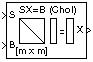# Cholesky Solver

Solve SX = B for X when S is a square Hermitian positive definite matrix

•Libraries:
DSP System Toolbox / Math Functions / Matrices and Linear Algebra / Linear System Solvers

## Description

The Cholesky Solver block solves the linear system SX = B by applying the Cholesky factorization to the input matrix, where:

• S is an M-by-M square matrix input through the S port. The matrix must be Hermitian positive definite.

• B is an M-by-N matrix input through the B port.

• X is the M-by-N output matrix and is the unique solution to the equations.

## Ports

### Input

expand all

Specify the input M-by-M square matrix S through this port. The matrix must be Hermitian positive definite. The block uses only the diagonal and upper triangle of the matrix and disregards any imaginary component in the diagonal entries. Inputs S and B must have the same number of rows.

Data Types: `single` | `double`
Complex Number Support: Yes

Specify the B matrix in the SX = B equation as an M-by-N matrix or an M-by-1 vector.

When you specify an M-by-1 vector, the block treats the length-M unoriented vector input at port B as an M-by-1 matrix. Inputs S and B must have the same number of rows.

Data Types: `single` | `double`
Complex Number Support: Yes

### Output

expand all

The block returns the output as an M-by-N matrix or an M-by-1 matrix. The size of the matrix X is same as the size of the matrix B.

Data Types: `single` | `double`
Complex Number Support: Yes

## Parameters

expand all

The input matrix S must be Hermitian positive definite.

When the input is not positive definite, the block reacts as per the behavior you specify in the Non-positive definite input parameter.

• `Ignore` — Proceed with the computation and do not issue an alert. The output is not a valid solution.

• `Warning` — Proceed with the computation and display a warning message in the MATLAB® command window. The output is not a valid solution.

• `Error` — Display an error dialog box and terminate the simulation.

Note

The Non-positive definite input parameter is a diagnostic parameter. Like all diagnostic parameters in the Configuration Parameters dialog box, it is set to `Ignore` in the code generated for this block by Simulink® Coder™ code generation software.

## Block Characteristics

 Data Types `double` | `single` Direct Feedthrough `no` Multidimensional Signals `no` Variable-Size Signals `no` Zero-Crossing Detection `no`

## Algorithms

Cholesky factorization uniquely factors the Hermitian positive definite input matrix S as

`$S=L{L}^{\ast }$`

where L is a lower triangular square matrix with positive diagonal elements.

The equation SX = B then becomes

`$L{L}^{\ast }X=B,$`

which is solved for X by substituting $Y={L}^{\ast }X$ and solving the following two triangular systems by forward and backward substitution, respectively.

`$LY=B$`
`${L}^{\ast }X=Y$`

## Version History

Introduced before R2006a